# Introduction

## Basics of ggmosaic

• designed to create visualizations of categorical data
• can produce bar charts, stacked bar charts, mosaic plots, and double decker plots
• plots are constructed hierarchically, so the ordering of the variables is very important.
• integrated in ggplot2 as a geom which allows for facetting and layering

## Creation of ggmosaic

ggmosaic was created primarily using ggproto and the productplots package

ggproto allows you to extend ggplot2 from within your own packages

• ggmosaic began as a geom extension of the rect geom
• used the data handling provided in the productplots package
• calculates xmin, xmax, ymin, and ymax for the rect geom to plot

## ggplot2 limitations

ggplot2 is not capable of handling a variable number of variables

• current solution: read in the variables x1 and x2 as x = product(x1, x2)

• product function:
• a wrapper function for a list
• allows for it to pass check_aesthetics

These limitations also lead to issues with the labeling, but those can be fixed manually.

## geom_mosaic: setting the aesthetics

Aesthetics that can be set:

• weight : select a weighting variable
• x : select variables to add to formula
• declared as x = product(x1, x2, …)
• fill : select a variable to be filled
• if the variable is not also called in x, it will be added to the formula in the first position
• conds : select a variable to condition on
• declared as conds = product(cond1, cond2, …)

These values are then sent through productplots functions to create the formula for the desired distribution

Formula: weight ~ fill + x | conds

### From the aesthetics to the formula

Example of how the formula is built

• weight = 1
• x = product(Y, X)
• fill = W
• conds = product(Z)

These aesthetics set up the formula for the distribution:

Formula: 1 ~ W + X + Y | Z

Because a mosaic plot is constructed hierarchically through alternating spines, the ordering of the variables is very important.

## 1 ~ X

 ggplot(data = fly) +
geom_mosaic(aes(x = product(RudeToRecline), fill=RudeToRecline), na.rm=TRUE) +
labs(x="Is it rude recline? ", title='f(RudeToRecline)')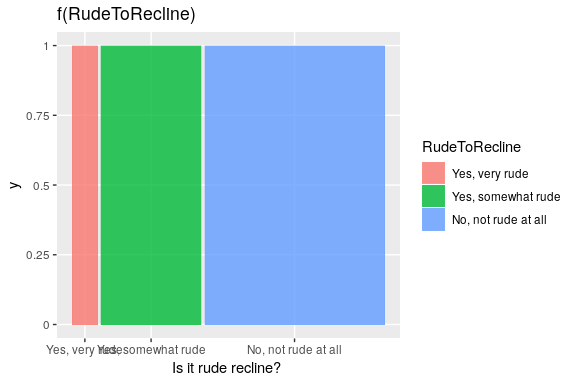## 1 ~ Y + X

ggplot(data = fly) +
geom_mosaic(aes(x = product(DoYouRecline, RudeToRecline), fill=DoYouRecline), na.rm=TRUE) +
labs(x = "Is it rude recline? ", title='f(DoYouRecline | RudeToRecline) f(RudeToRecline)')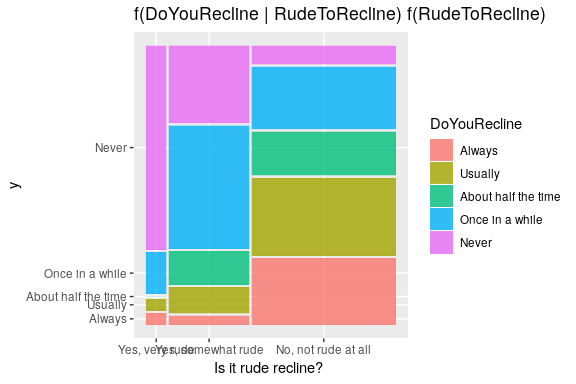## 1 ~ X + Y / Z

ggplot(data = fly) +
geom_mosaic(aes(x = product(DoYouRecline, RudeToRecline), fill=DoYouRecline, conds=product(Gender)), na.rm=TRUE, divider=mosaic("v")) +  labs(x = "Is it rude recline? ", title='f(DoYouRecline, RudeToRecline| Gender)')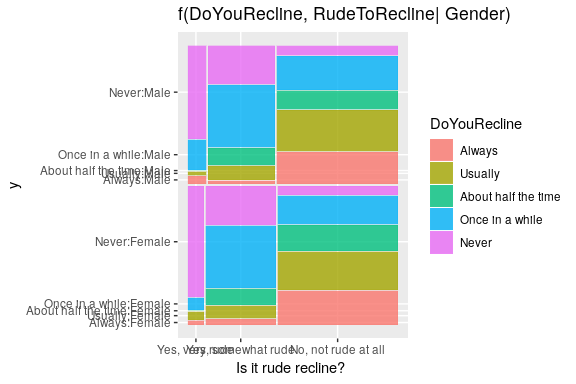## Alternative to conditioning: facetting

ggplot(data = fly) +
geom_mosaic(aes(x = product(DoYouRecline, RudeToRecline), fill=DoYouRecline), na.rm=TRUE) +  labs(x = "Is it rude recline? ", title='f(DoYouRecline, RudeToRecline| Gender)') + facet_grid(Gender~.)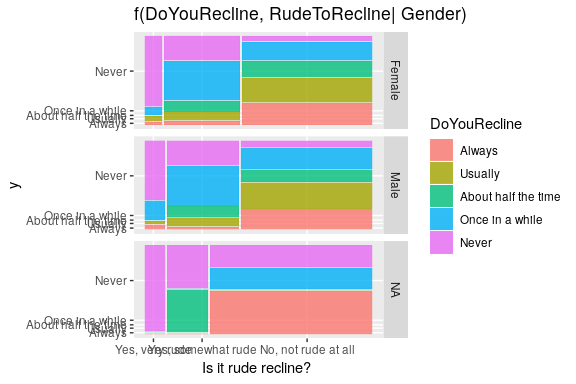## Importance of ordering

order1 <- ggplot(data = fly) +
geom_mosaic(aes(x = product(DoYouRecline, RudeToRecline), fill=DoYouRecline), na.rm=TRUE) +  labs(x = "Is it rude recline? ", title='f(DoYouRecline | RudeToRecline) f(RudeToRecline)') + theme(plot.title = element_text(size = rel(1)))

order2<- ggplot(data = fly) +
geom_mosaic(aes(x = product(RudeToRecline, DoYouRecline), fill=DoYouRecline), na.rm=TRUE) + labs(x = "" , y = "Is it rude recline? ", title='f(DoYouRecline | RudeToRecline) f(RudeToRecline)') + coord_flip() + theme(plot.title = element_text(size = rel(1)))
grid_arrange_shared_legend(order1, order2, ncol = 2, nrow = 1, position = "right")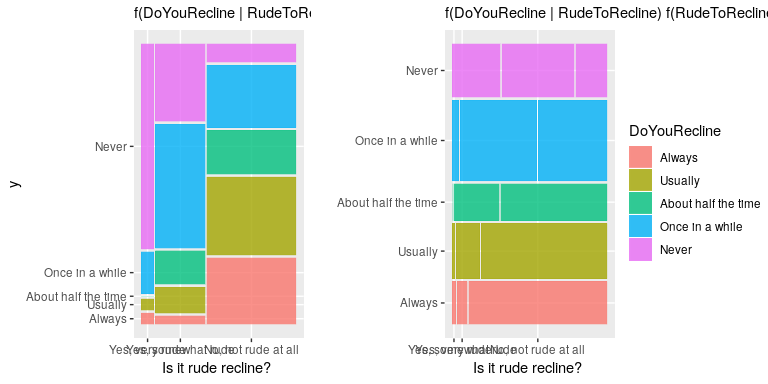## Other features of geom_mosaic

Arguments unique to geom_mosaic:

• divider: used to declare the type of partitions to be used
• offset: sets the space between the first spine

## Divider function: Types of partitioning

Four options available for each partion:

• vspine: width constant, height varies.
• hspine: height constant, width varies.
• vbar: height constant, width varies.
• hbar: width constant, height varies.
hbar <- ggplot(data = fly) +
geom_mosaic(aes(x = product(FlightFreq), fill=FlightFreq), divider="hbar", na.rm=TRUE) + labs(x=" ", title='divider = "hbar"')

hspine <- ggplot(data = fly) +
geom_mosaic(aes(x = product(FlightFreq), fill=FlightFreq),  divider="hspine", na.rm=TRUE) + labs(x=" ", title='divider = "hspine"')

vbar <- ggplot(data = fly) +
geom_mosaic(aes(x = product(FlightFreq), fill=FlightFreq), divider="vbar", na.rm=TRUE) + labs(y=" ", x="", title='divider = "vbar"')

vspine <- ggplot(data = fly) +
geom_mosaic(aes(x = product(FlightFreq), fill=FlightFreq), divider="vspine", na.rm=TRUE) + labs(y=" ", x="", title='divider = "vspine"') 
grid_arrange_shared_legend(hbar, hspine, vbar, vspine, ncol = 2, nrow = 2, position = "right")

## Partitioning with one or more variables

• mosaic()
• default
• will use spines in alternating directions
• begins with a horizontal spine
• mosaic(“v”)
• begins with a vertical spine and then alternates
• ddecker()
• selects n-1 horizontal spines and ends with a vertical spine
• Define each type of partition
• c(“hspine”, “vspine”, “hbar”)
h_mosaic <- ggplot(data = fly) +
geom_mosaic(aes(x = product(FlightFreq, Gender, Region), fill=FlightFreq), na.rm=T, divider=mosaic("h")) +
theme(axis.text.x=element_blank(), legend.position="none") +
labs(x=" ", title='divider= mosaic()')

v_mosaic <- ggplot(data = fly) +
geom_mosaic(aes(x = product(FlightFreq, Gender, Region), fill=FlightFreq), na.rm=T, divider=mosaic("v")) +
theme(axis.text.x=element_blank()) +
labs(x=" ", title='divider= mosaic("v")')

doubledecker <- ggplot(data = fly) +
geom_mosaic(aes(x = product(FlightFreq, Gender, Region), fill=FlightFreq), na.rm=T, divider=ddecker()) +
theme(axis.text.x=element_blank()) +
labs(x=" ", title='divider= ddecker()')
grid_arrange_shared_legend(h_mosaic, v_mosaic, doubledecker, ncol = 3, nrow = 1, position = "right")
mosaic4 <-  ggplot(data = fly) +
geom_mosaic(aes(x = product(FlightFreq, Gender, Region), fill=FlightFreq), na.rm=T, divider=c("vspine", "vspine", "hbar")) +
theme(axis.text.x=element_blank()) +
labs(x=" ", title='divider= c("vspine", "vspine", "hbar")')

mosaic5 <-  ggplot(data = fly) +
geom_mosaic(aes(x = product(FlightFreq, Gender, Region), fill=FlightFreq), na.rm=T, divider=c("hbar", "vspine", "hbar")) +
theme(axis.text.x=element_blank()) +
labs(x=" ", title='divider= c("hbar", "vspine", "hbar")')

mosaic6 <-  ggplot(data = fly) +
geom_mosaic(aes(x = product(FlightFreq, Gender, Region), fill=FlightFreq), na.rm=T, divider=c("hspine", "hspine", "hspine")) +
theme(axis.text.x=element_blank()) +
labs(x=" ", title='divider= c("hspine", "hspine", "hspine")')

mosaic7 <-  ggplot(data = fly) +
geom_mosaic(aes(x = product(FlightFreq, Gender, Region), fill=FlightFreq), na.rm=T, divider=c("vspine", "vspine", "vspine")) +
theme(axis.text.x=element_blank()) +
labs(x=" ", title='divider= c("vspine", "vspine", "vspine")')
grid_arrange_shared_legend(mosaic4, mosaic5, mosaic6, mosaic7, ncol = 2, nrow = 2, position="right")

## geom_mosaic: offset

offset: Set the space between the first spine

• default = 0.01
• space between partitions decreases as layers build

## Adjusting the offset

offset1 <- ggplot(data = fly) +
geom_mosaic(aes(x = product(FlightFreq, Region), fill=FlightFreq), na.rm=TRUE) + labs(x="Region", y=" ",  title=" offset = 0.01")

offset0 <- ggplot(data = fly) +
geom_mosaic(aes(x = product(FlightFreq, Region), fill=FlightFreq), na.rm=TRUE, offset = 0) + labs(x="Region", y=" ",  title=" offset = 0")

offset2 <- ggplot(data = fly) +
geom_mosaic(aes(x = product(FlightFreq, Region), fill=FlightFreq), na.rm=TRUE, offset = 0.02) + labs(x="Region", y=" ",  title=" offset = 0.02")
grid_arrange_shared_legend(offset0, offset1, offset2, nrow = 1, ncol =3, position="right")

## Plotly

gg <-ggplot(data = fly) +
geom_mosaic(aes(x = product(DoYouRecline, RudeToRecline), fill=DoYouRecline), na.rm=TRUE) + labs(x = "Is it rude recline? ", title='f(DoYouRecline | RudeToRecline) f(RudeToRecline)')
# just for now commented out
# ggplotly(gg)